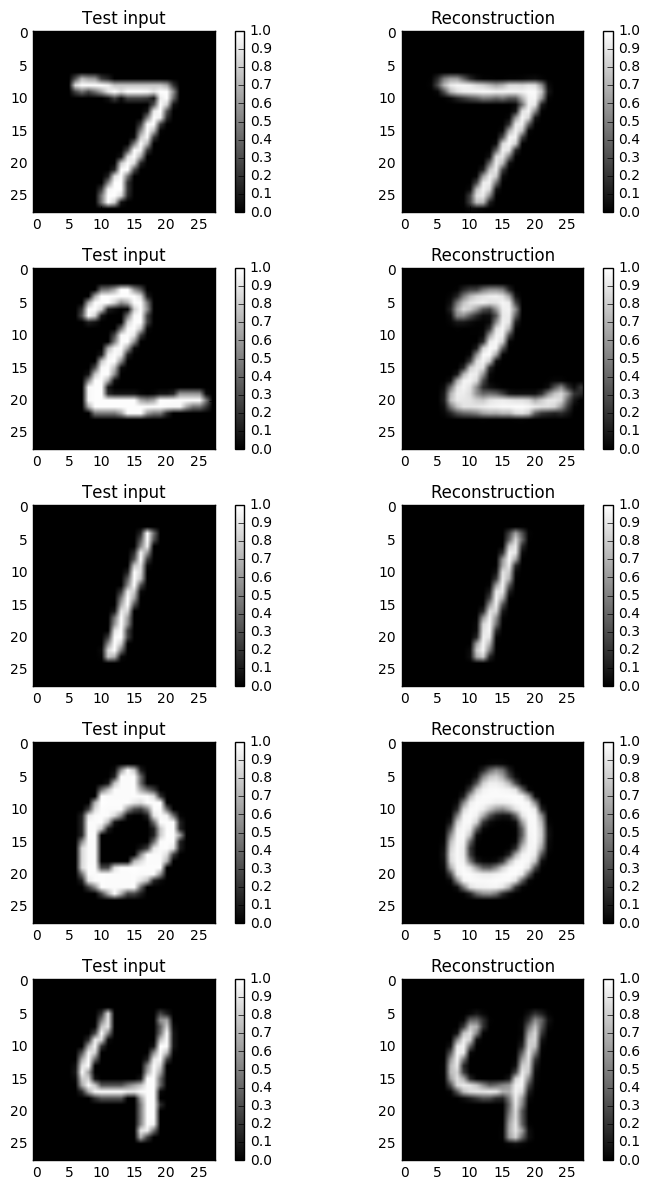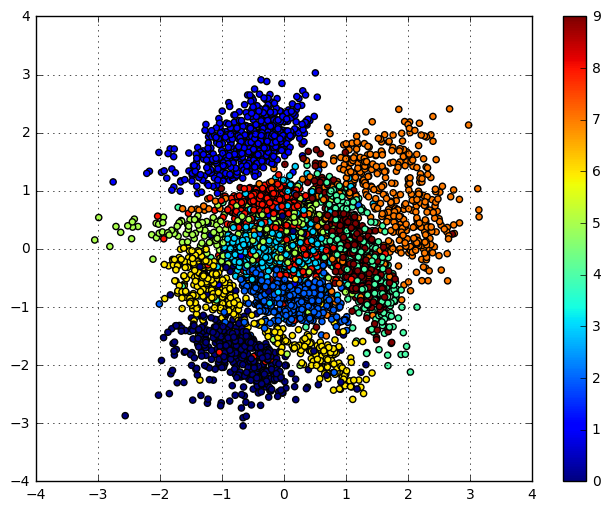# Variational Autoencoder in TensorFlow¶

The main motivation for this post was that I wanted to get more experience with both Variational Autoencoders (VAEs) and with Tensorflow. Thus, implementing the former in the latter sounded like a good idea for learning about both at the same time. This post summarizes the result.

Note: The post was updated on December 7th 2015:

• a bug in the computation of the latent_loss was fixed (removed an erroneous factor 2). Thanks Colin Fang for pointing this out.
• Using a Bernoulli distribution rather than a Gaussian distribution in the generator network

Note: The post was updated on January 3rd 2017:

• changes required for supporting TensorFlow v0.12 and Python 3 support

Let us first do the necessary imports, load the data (MNIST), and define some helper functions.

In :
import numpy as np
import tensorflow as tf

import matplotlib.pyplot as plt
%matplotlib inline

np.random.seed(0)
tf.set_random_seed(0)

In :
# Load MNIST data in a format suited for tensorflow.
# The script input_data is available under this URL:
# https://raw.githubusercontent.com/tensorflow/tensorflow/master/tensorflow/examples/tutorials/mnist/input_data.py
import input_data
mnist = input_data.read_data_sets('MNIST_data', one_hot=True)
n_samples = mnist.train.num_examples

Extracting MNIST_data/train-images-idx3-ubyte.gz
Extracting MNIST_data/train-labels-idx1-ubyte.gz
Extracting MNIST_data/t10k-images-idx3-ubyte.gz
Extracting MNIST_data/t10k-labels-idx1-ubyte.gz

In :
def xavier_init(fan_in, fan_out, constant=1):
""" Xavier initialization of network weights"""
# https://stackoverflow.com/questions/33640581/how-to-do-xavier-initialization-on-tensorflow
low = -constant*np.sqrt(6.0/(fan_in + fan_out))
high = constant*np.sqrt(6.0/(fan_in + fan_out))
return tf.random_uniform((fan_in, fan_out),
minval=low, maxval=high,
dtype=tf.float32)


Based on this, we define now a class "VariationalAutoencoder" with a sklearn-like interface that can be trained incrementally with mini-batches using partial_fit. The trained model can be used to reconstruct unseen input, to generate new samples, and to map inputs to the latent space.

In :
class VariationalAutoencoder(object):
""" Variation Autoencoder (VAE) with an sklearn-like interface implemented using TensorFlow.

This implementation uses probabilistic encoders and decoders using Gaussian
distributions and  realized by multi-layer perceptrons. The VAE can be learned
end-to-end.

See "Auto-Encoding Variational Bayes" by Kingma and Welling for more details.
"""
def __init__(self, network_architecture, transfer_fct=tf.nn.softplus,
learning_rate=0.001, batch_size=100):
self.network_architecture = network_architecture
self.transfer_fct = transfer_fct
self.learning_rate = learning_rate
self.batch_size = batch_size

# tf Graph input
self.x = tf.placeholder(tf.float32, [None, network_architecture["n_input"]])

# Create autoencoder network
self._create_network()
# Define loss function based variational upper-bound and
# corresponding optimizer
self._create_loss_optimizer()

# Initializing the tensor flow variables
init = tf.global_variables_initializer()

# Launch the session
self.sess = tf.InteractiveSession()
self.sess.run(init)

def _create_network(self):
# Initialize autoencode network weights and biases
network_weights = self._initialize_weights(**self.network_architecture)

# Use recognition network to determine mean and
# (log) variance of Gaussian distribution in latent
# space
self.z_mean, self.z_log_sigma_sq = \
self._recognition_network(network_weights["weights_recog"],
network_weights["biases_recog"])

# Draw one sample z from Gaussian distribution
n_z = self.network_architecture["n_z"]
eps = tf.random_normal((self.batch_size, n_z), 0, 1,
dtype=tf.float32)
# z = mu + sigma*epsilon
self.z = tf.add(self.z_mean,
tf.mul(tf.sqrt(tf.exp(self.z_log_sigma_sq)), eps))

# Use generator to determine mean of
# Bernoulli distribution of reconstructed input
self.x_reconstr_mean = \
self._generator_network(network_weights["weights_gener"],
network_weights["biases_gener"])

def _initialize_weights(self, n_hidden_recog_1, n_hidden_recog_2,
n_hidden_gener_1,  n_hidden_gener_2,
n_input, n_z):
all_weights = dict()
all_weights['weights_recog'] = {
'h1': tf.Variable(xavier_init(n_input, n_hidden_recog_1)),
'h2': tf.Variable(xavier_init(n_hidden_recog_1, n_hidden_recog_2)),
'out_mean': tf.Variable(xavier_init(n_hidden_recog_2, n_z)),
'out_log_sigma': tf.Variable(xavier_init(n_hidden_recog_2, n_z))}
all_weights['biases_recog'] = {
'b1': tf.Variable(tf.zeros([n_hidden_recog_1], dtype=tf.float32)),
'b2': tf.Variable(tf.zeros([n_hidden_recog_2], dtype=tf.float32)),
'out_mean': tf.Variable(tf.zeros([n_z], dtype=tf.float32)),
'out_log_sigma': tf.Variable(tf.zeros([n_z], dtype=tf.float32))}
all_weights['weights_gener'] = {
'h1': tf.Variable(xavier_init(n_z, n_hidden_gener_1)),
'h2': tf.Variable(xavier_init(n_hidden_gener_1, n_hidden_gener_2)),
'out_mean': tf.Variable(xavier_init(n_hidden_gener_2, n_input)),
'out_log_sigma': tf.Variable(xavier_init(n_hidden_gener_2, n_input))}
all_weights['biases_gener'] = {
'b1': tf.Variable(tf.zeros([n_hidden_gener_1], dtype=tf.float32)),
'b2': tf.Variable(tf.zeros([n_hidden_gener_2], dtype=tf.float32)),
'out_mean': tf.Variable(tf.zeros([n_input], dtype=tf.float32)),
'out_log_sigma': tf.Variable(tf.zeros([n_input], dtype=tf.float32))}
return all_weights

def _recognition_network(self, weights, biases):
# Generate probabilistic encoder (recognition network), which
# maps inputs onto a normal distribution in latent space.
# The transformation is parametrized and can be learned.
layer_1 = self.transfer_fct(tf.add(tf.matmul(self.x, weights['h1']),
biases['b1']))
layer_2 = self.transfer_fct(tf.add(tf.matmul(layer_1, weights['h2']),
biases['b2']))
z_mean = tf.add(tf.matmul(layer_2, weights['out_mean']),
biases['out_mean'])
z_log_sigma_sq = \
tf.add(tf.matmul(layer_2, weights['out_log_sigma']),
biases['out_log_sigma'])
return (z_mean, z_log_sigma_sq)

def _generator_network(self, weights, biases):
# Generate probabilistic decoder (decoder network), which
# maps points in latent space onto a Bernoulli distribution in data space.
# The transformation is parametrized and can be learned.
layer_1 = self.transfer_fct(tf.add(tf.matmul(self.z, weights['h1']),
biases['b1']))
layer_2 = self.transfer_fct(tf.add(tf.matmul(layer_1, weights['h2']),
biases['b2']))
x_reconstr_mean = \
tf.nn.sigmoid(tf.add(tf.matmul(layer_2, weights['out_mean']),
biases['out_mean']))
return x_reconstr_mean

def _create_loss_optimizer(self):
# The loss is composed of two terms:
# 1.) The reconstruction loss (the negative log probability
#     of the input under the reconstructed Bernoulli distribution
#     induced by the decoder in the data space).
#     This can be interpreted as the number of "nats" required
#     for reconstructing the input when the activation in latent
#     is given.
# Adding 1e-10 to avoid evaluation of log(0.0)
reconstr_loss = \
-tf.reduce_sum(self.x * tf.log(1e-10 + self.x_reconstr_mean)
+ (1-self.x) * tf.log(1e-10 + 1 - self.x_reconstr_mean),
1)
# 2.) The latent loss, which is defined as the Kullback Leibler divergence
##    between the distribution in latent space induced by the encoder on
#     the data and some prior. This acts as a kind of regularizer.
#     This can be interpreted as the number of "nats" required
#     for transmitting the the latent space distribution given
#     the prior.
latent_loss = -0.5 * tf.reduce_sum(1 + self.z_log_sigma_sq
- tf.square(self.z_mean)
- tf.exp(self.z_log_sigma_sq), 1)
self.cost = tf.reduce_mean(reconstr_loss + latent_loss)   # average over batch
# Use ADAM optimizer
self.optimizer = \
tf.train.AdamOptimizer(learning_rate=self.learning_rate).minimize(self.cost)

def partial_fit(self, X):
"""Train model based on mini-batch of input data.

Return cost of mini-batch.
"""
opt, cost = self.sess.run((self.optimizer, self.cost),
feed_dict={self.x: X})
return cost

def transform(self, X):
"""Transform data by mapping it into the latent space."""
# Note: This maps to mean of distribution, we could alternatively
# sample from Gaussian distribution
return self.sess.run(self.z_mean, feed_dict={self.x: X})

def generate(self, z_mu=None):
""" Generate data by sampling from latent space.

If z_mu is not None, data for this point in latent space is
generated. Otherwise, z_mu is drawn from prior in latent
space.
"""
if z_mu is None:
z_mu = np.random.normal(size=self.network_architecture["n_z"])
# Note: This maps to mean of distribution, we could alternatively
# sample from Gaussian distribution
return self.sess.run(self.x_reconstr_mean,
feed_dict={self.z: z_mu})

def reconstruct(self, X):
""" Use VAE to reconstruct given data. """
return self.sess.run(self.x_reconstr_mean,
feed_dict={self.x: X})


In general, implementing a VAE in tensorflow is relatively straightforward (in particular since we don not need to code the gradient computation). A bit confusing is potentially that all the logic happens at initialization of the class (where the graph is generated), while the actual sklearn interface methods are very simple one-liners.

We can now define a simple fuction which trains the VAE using mini-batches:

In :
def train(network_architecture, learning_rate=0.001,
batch_size=100, training_epochs=10, display_step=5):
vae = VariationalAutoencoder(network_architecture,
learning_rate=learning_rate,
batch_size=batch_size)
# Training cycle
for epoch in range(training_epochs):
avg_cost = 0.
total_batch = int(n_samples / batch_size)
# Loop over all batches
for i in range(total_batch):
batch_xs, _ = mnist.train.next_batch(batch_size)

# Fit training using batch data
cost = vae.partial_fit(batch_xs)
# Compute average loss
avg_cost += cost / n_samples * batch_size

# Display logs per epoch step
if epoch % display_step == 0:
print("Epoch:", '%04d' % (epoch+1),
"cost=", "{:.9f}".format(avg_cost))
return vae


## Illustrating reconstruction quality¶

We can now train a VAE on MNIST by just specifying the network topology. We start with training a VAE with a 20-dimensional latent space.

In :
network_architecture = \
dict(n_hidden_recog_1=500, # 1st layer encoder neurons
n_hidden_recog_2=500, # 2nd layer encoder neurons
n_hidden_gener_1=500, # 1st layer decoder neurons
n_hidden_gener_2=500, # 2nd layer decoder neurons
n_input=784, # MNIST data input (img shape: 28*28)
n_z=20)  # dimensionality of latent space

vae = train(network_architecture, training_epochs=75)

Epoch: 0001 cost= 181.437451200
Epoch: 0006 cost= 110.449812497
Epoch: 0011 cost= 106.030304274
Epoch: 0016 cost= 103.363558572
Epoch: 0021 cost= 101.201389451
Epoch: 0026 cost= 99.697997270
Epoch: 0031 cost= 98.750394565
Epoch: 0036 cost= 97.979149531
Epoch: 0041 cost= 97.337145219
Epoch: 0046 cost= 96.880868322
Epoch: 0051 cost= 96.415262562
Epoch: 0056 cost= 96.061289187
Epoch: 0061 cost= 95.761952903
Epoch: 0066 cost= 95.480659388
Epoch: 0071 cost= 95.174087233


Based on this we can sample some test inputs and visualize how well the VAE can reconstruct those. In general the VAE does really well.

In :
x_sample = mnist.test.next_batch(100)
x_reconstruct = vae.reconstruct(x_sample)

plt.figure(figsize=(8, 12))
for i in range(5):

plt.subplot(5, 2, 2*i + 1)
plt.imshow(x_sample[i].reshape(28, 28), vmin=0, vmax=1, cmap="gray")
plt.title("Test input")
plt.colorbar()
plt.subplot(5, 2, 2*i + 2)
plt.imshow(x_reconstruct[i].reshape(28, 28), vmin=0, vmax=1, cmap="gray")
plt.title("Reconstruction")
plt.colorbar()
plt.tight_layout()## Illustrating latent space¶

Next, we train a VAE with 2d latent space and illustrates how the encoder (the recognition network) encodes some of the labeled inputs (collapsing the Gaussian distribution in latent space to its mean). This gives us some insights into the structure of the learned manifold (latent space)

In :
network_architecture = \
dict(n_hidden_recog_1=500, # 1st layer encoder neurons
n_hidden_recog_2=500, # 2nd layer encoder neurons
n_hidden_gener_1=500, # 1st layer decoder neurons
n_hidden_gener_2=500, # 2nd layer decoder neurons
n_input=784, # MNIST data input (img shape: 28*28)
n_z=2)  # dimensionality of latent space

vae_2d = train(network_architecture, training_epochs=75)

Epoch: 0001 cost= 187.989159047
Epoch: 0006 cost= 153.522320945
Epoch: 0011 cost= 148.390766352
Epoch: 0016 cost= 145.729972423
Epoch: 0021 cost= 144.193653717
Epoch: 0026 cost= 143.046030051
Epoch: 0031 cost= 142.209316600
Epoch: 0036 cost= 141.562141529
Epoch: 0041 cost= 140.994664584
Epoch: 0046 cost= 140.519218500
Epoch: 0051 cost= 140.079906838
Epoch: 0056 cost= 139.805822754
Epoch: 0061 cost= 139.486802576
Epoch: 0066 cost= 139.185289986
Epoch: 0071 cost= 138.955365503

In :
x_sample, y_sample = mnist.test.next_batch(5000)
z_mu = vae_2d.transform(x_sample)
plt.figure(figsize=(8, 6))
plt.scatter(z_mu[:, 0], z_mu[:, 1], c=np.argmax(y_sample, 1))
plt.colorbar()
plt.grid()An other way of getting insights into the latent space is to use the generator network to plot reconstrunctions at the positions in the latent space for which they have been generated:

In :
nx = ny = 20
x_values = np.linspace(-3, 3, nx)
y_values = np.linspace(-3, 3, ny)

canvas = np.empty((28*ny, 28*nx))
for i, yi in enumerate(x_values):
for j, xi in enumerate(y_values):
z_mu = np.array([[xi, yi]]*vae.batch_size)
x_mean = vae_2d.generate(z_mu)
canvas[(nx-i-1)*28:(nx-i)*28, j*28:(j+1)*28] = x_mean.reshape(28, 28)

plt.figure(figsize=(8, 10))
Xi, Yi = np.meshgrid(x_values, y_values)
plt.imshow(canvas, origin="upper", cmap="gray")
plt.tight_layout()